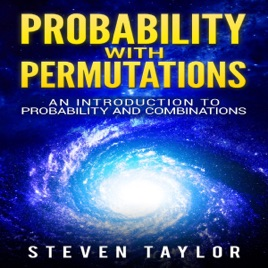• \$5.99

## Publisher Description

Understanding probability as unique and stimulating theory which goes beyond conventional mathematics will give you better perspective of the world around you. The first part of the book explains the fundamentals of probability in a clear and easy-to-understand way even if you are not familiar with mathematics at all, and you are just starting your journey towards this particular field of science. In the following sections of the book, the subject is explained in wider context along with importance of permutations and combinations in probability and their applications to a variety of scientific problems, as well as the importance of probability in real life situations.

By listening to this book now, you will discover:

History of probability
Explanation of combinations
Probability using permutations and combinations
Urn problems
Probability and lottery
Probability and gambling
Applications of probability
And much, much more!